# 【Lecture 20】Nonlinear Structured Models: Transform Invariant Low-Rank Texture.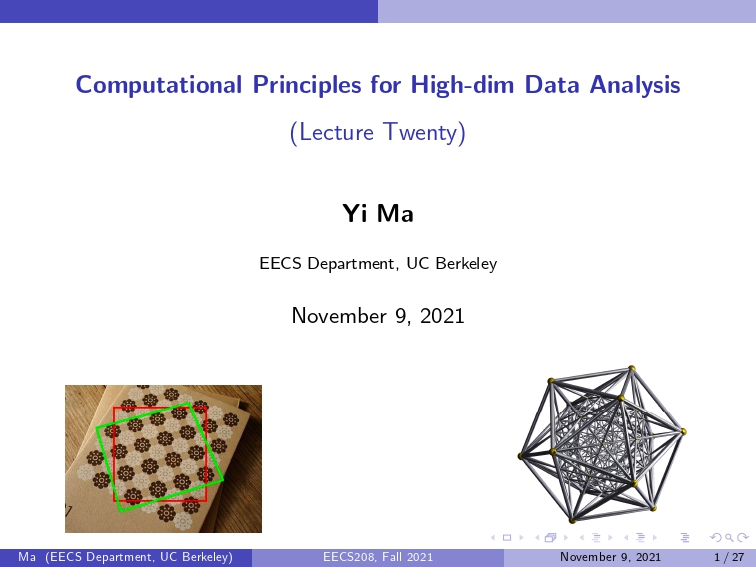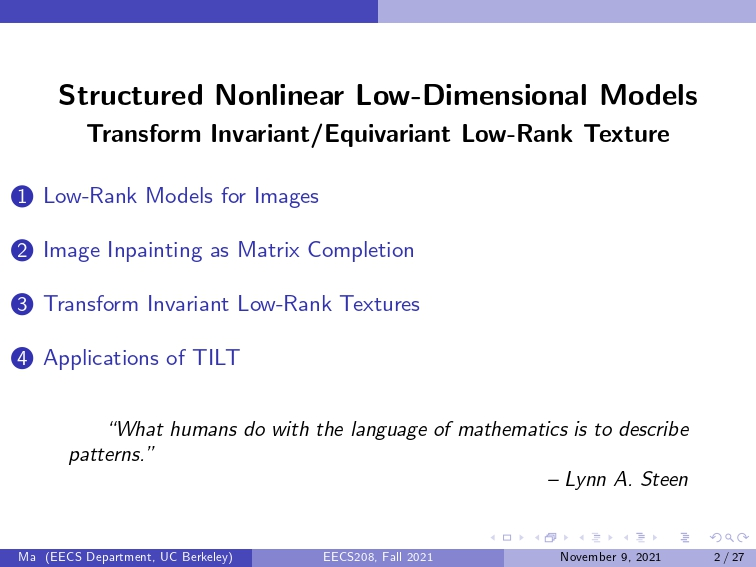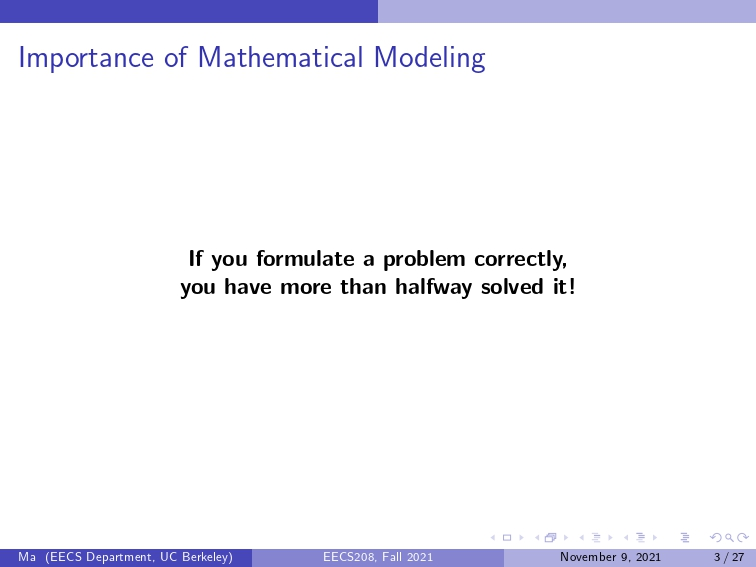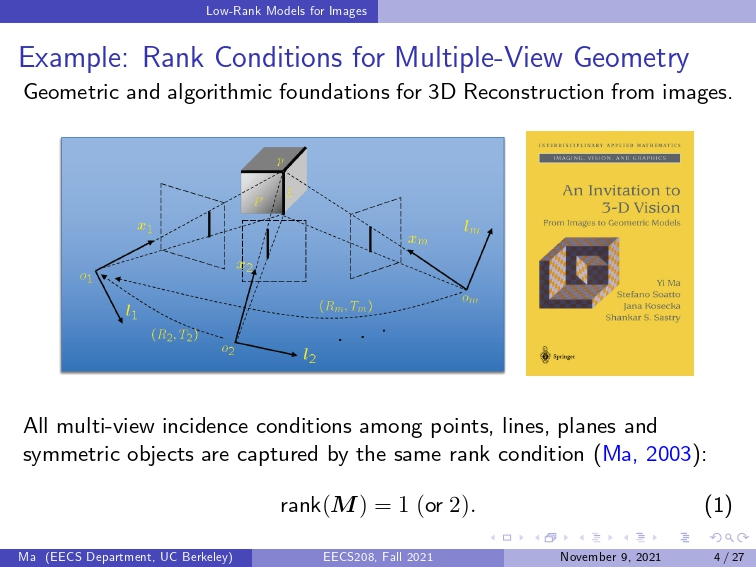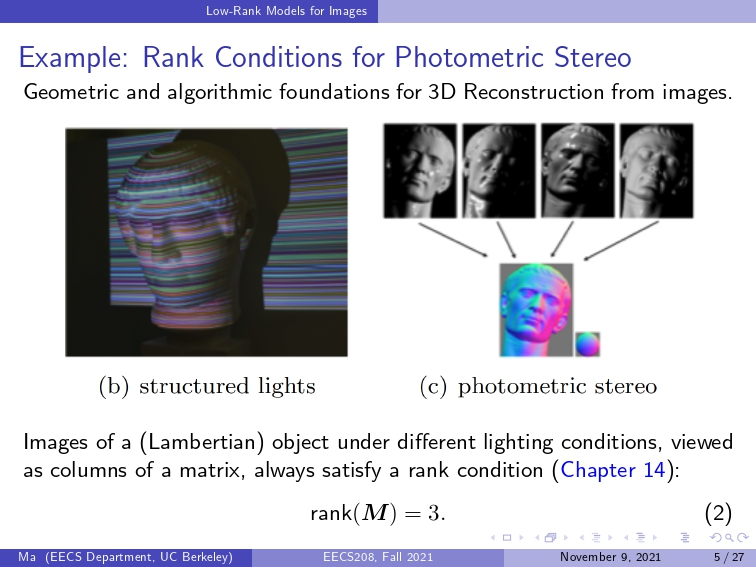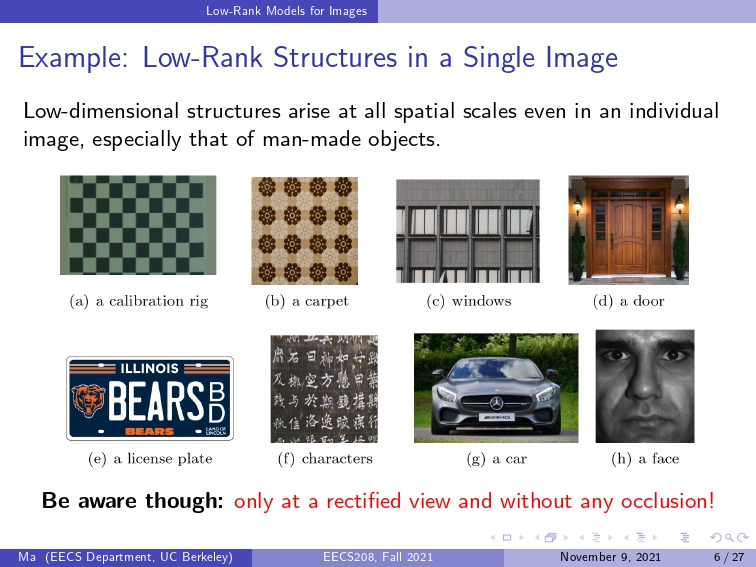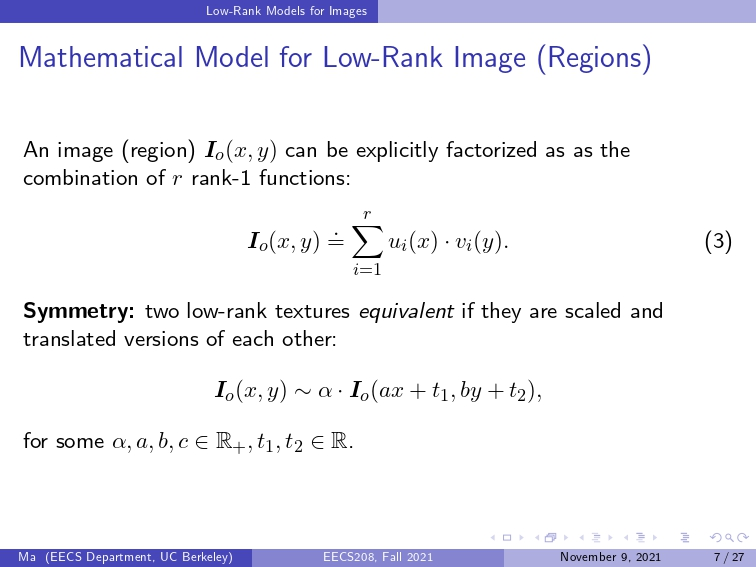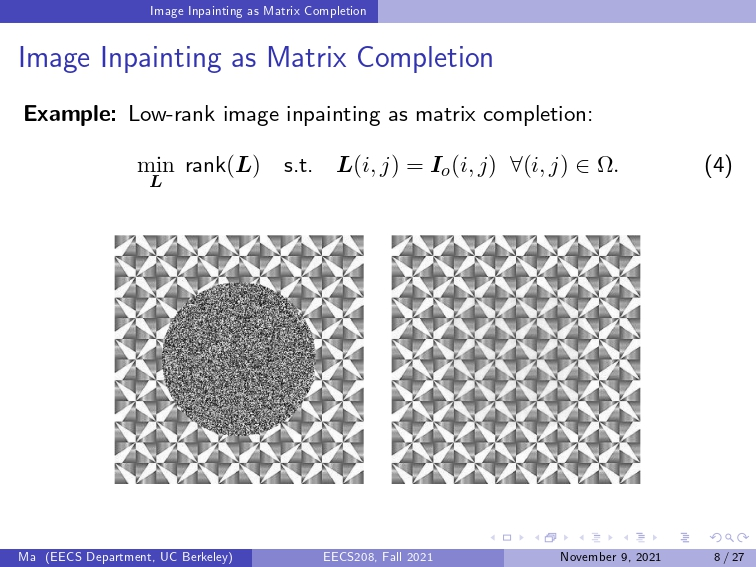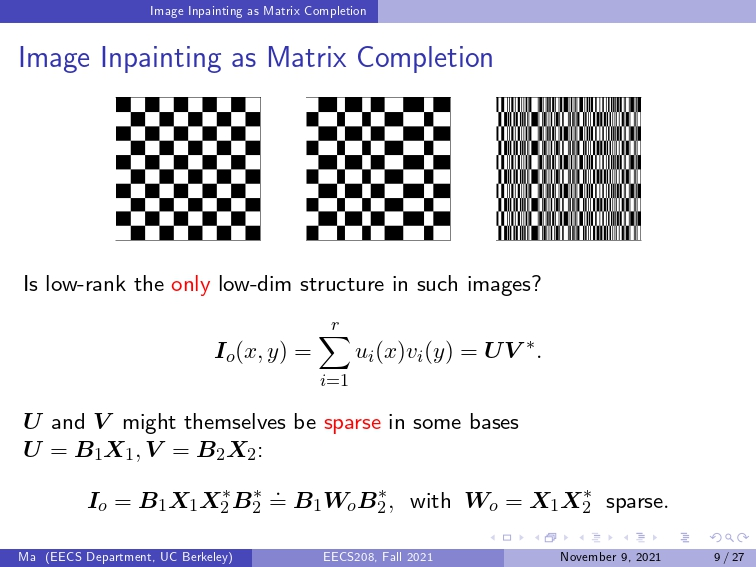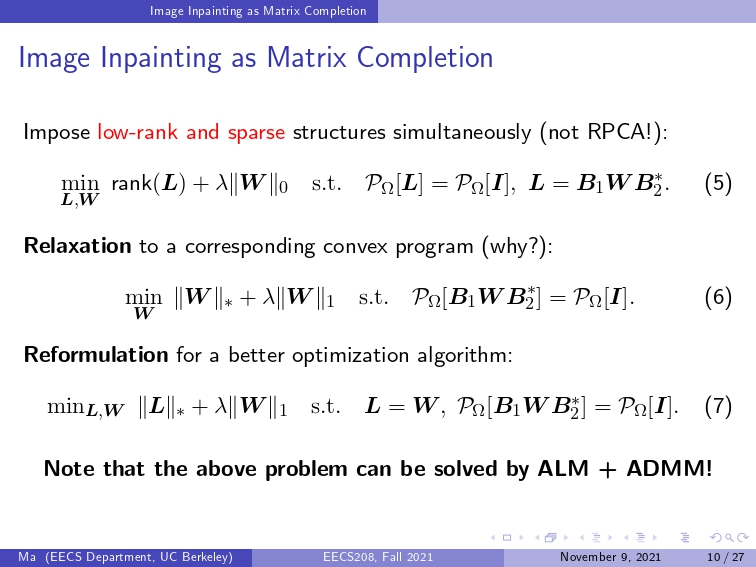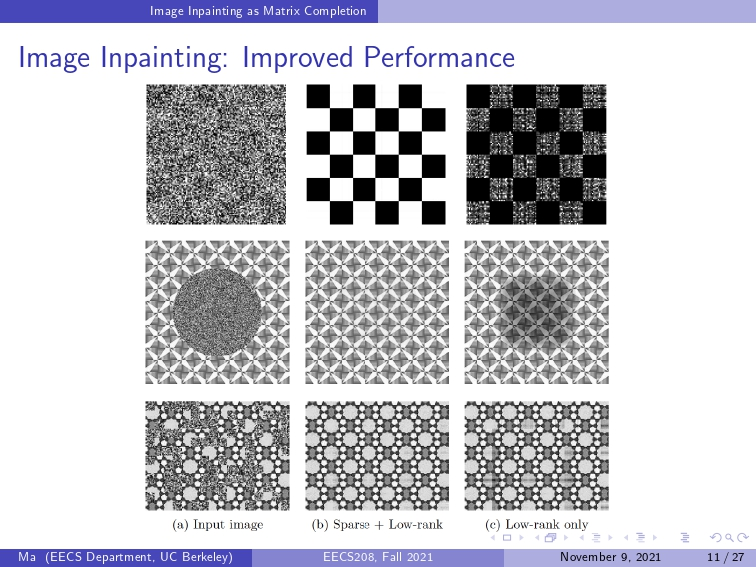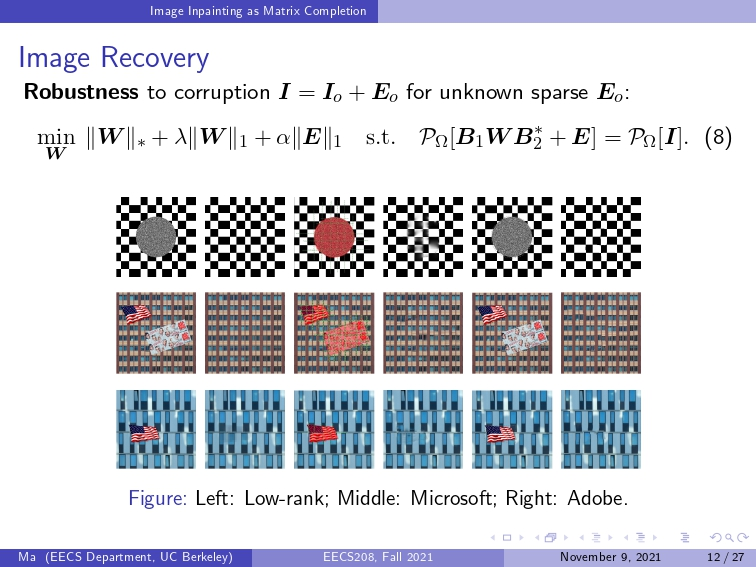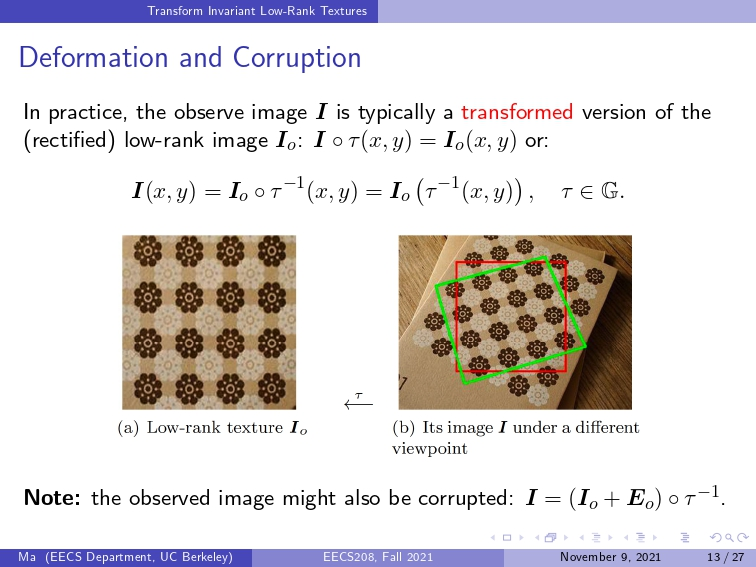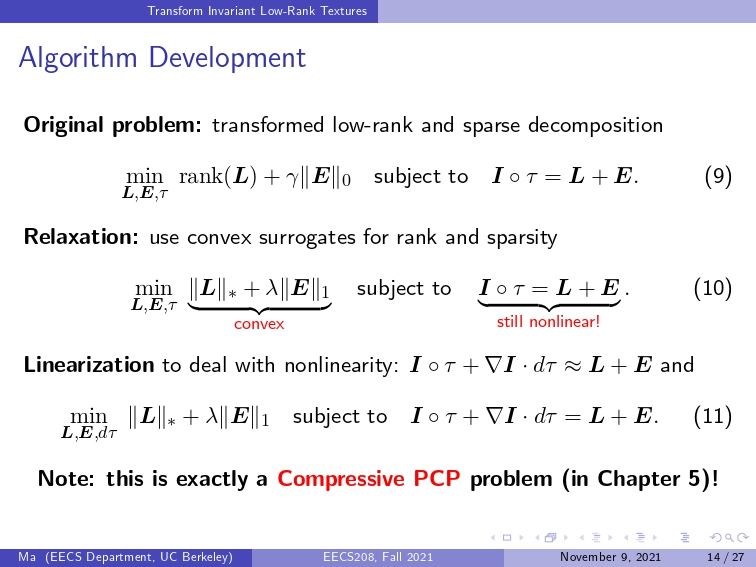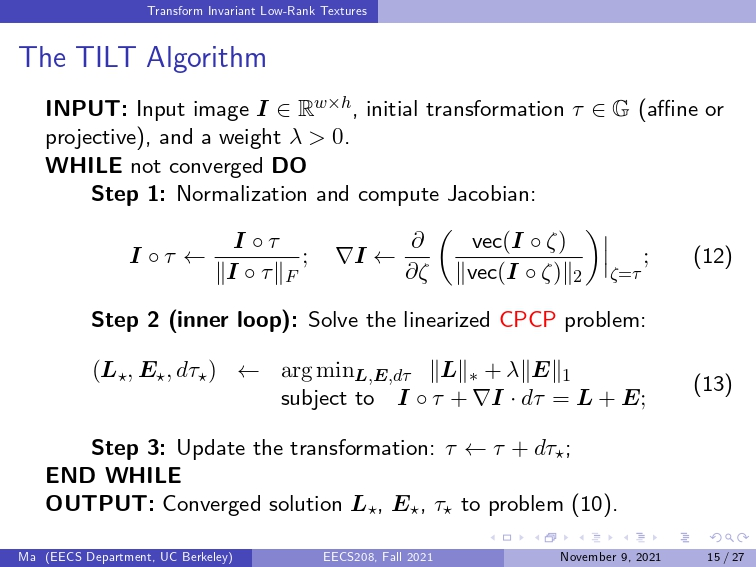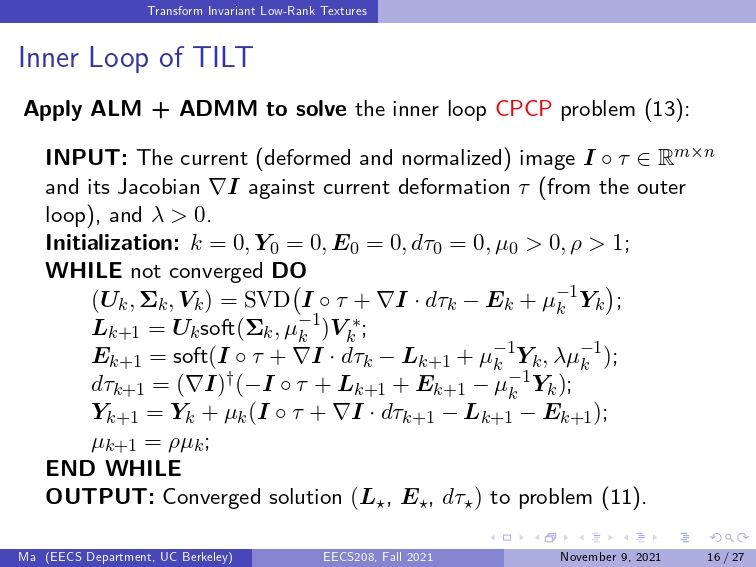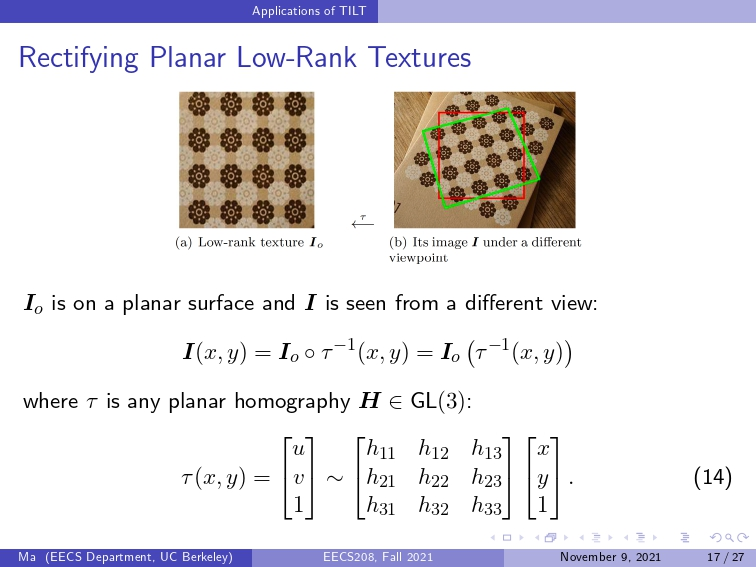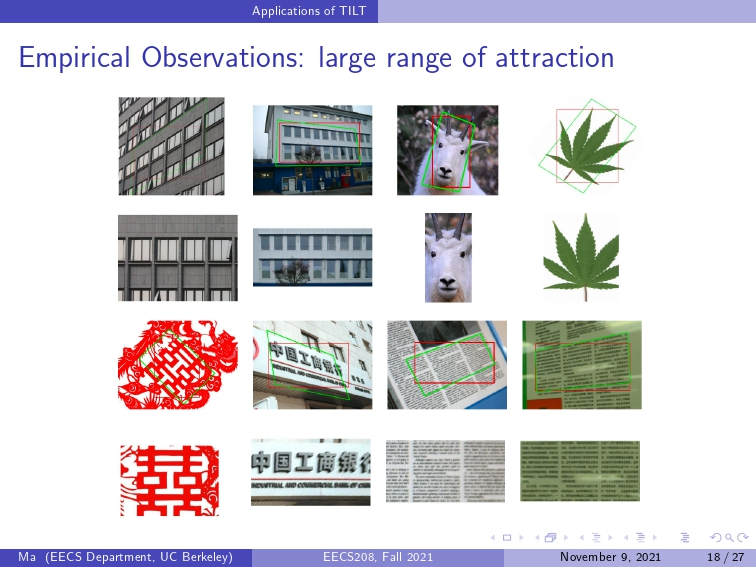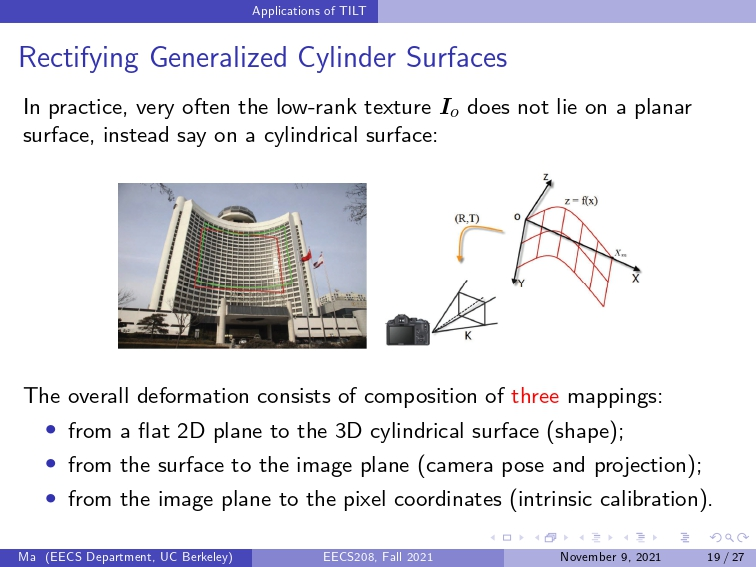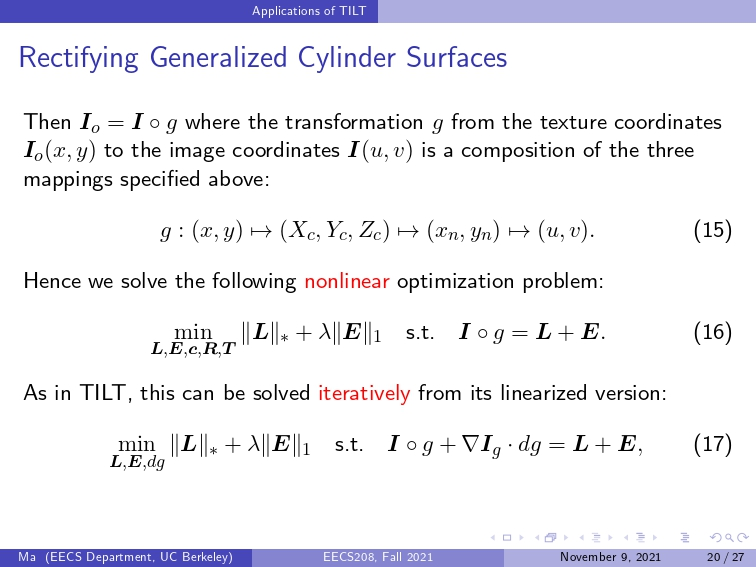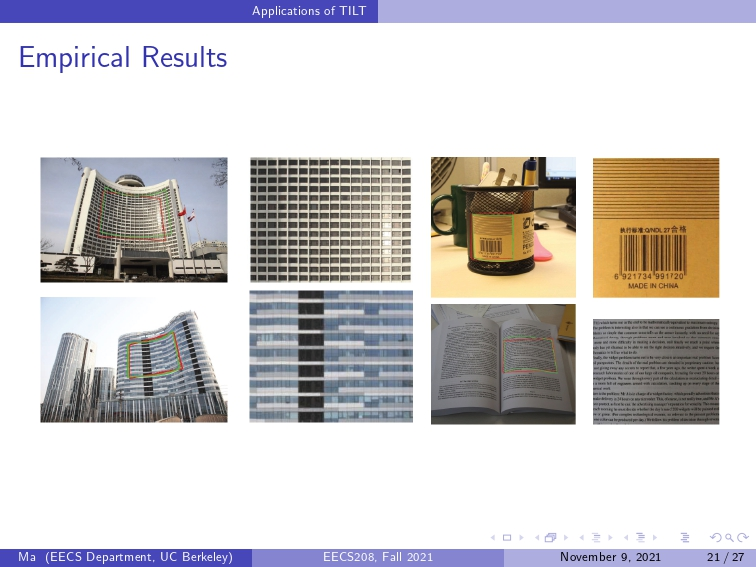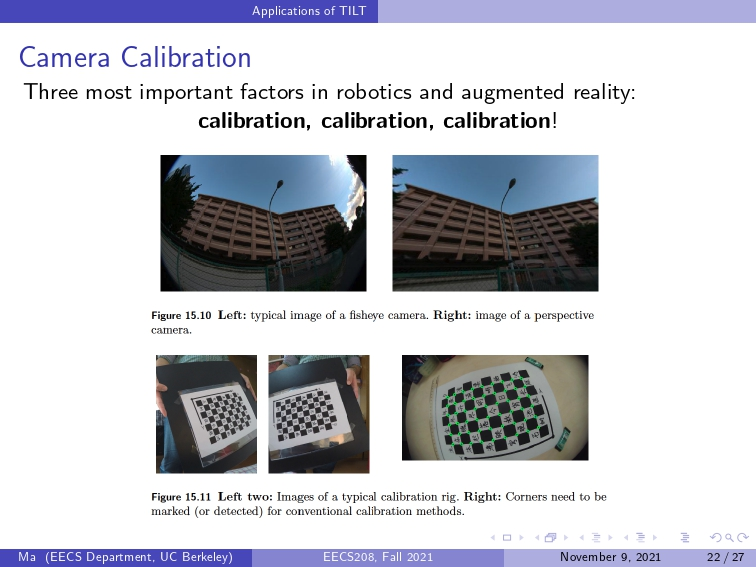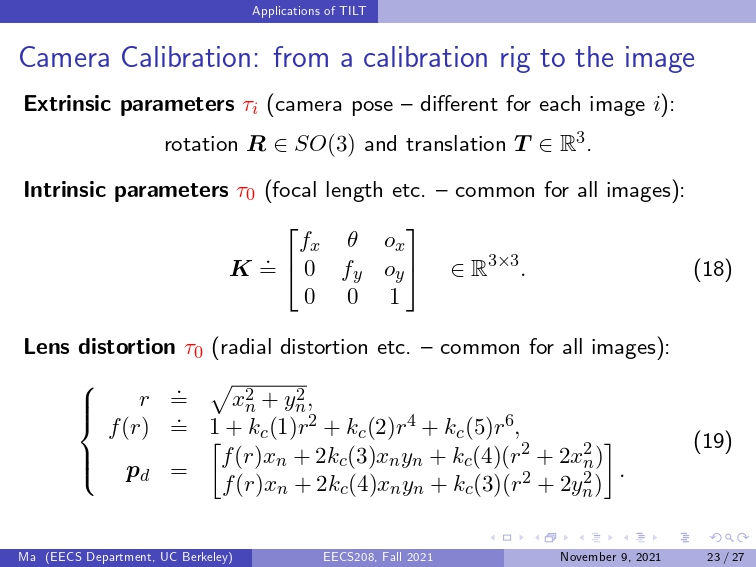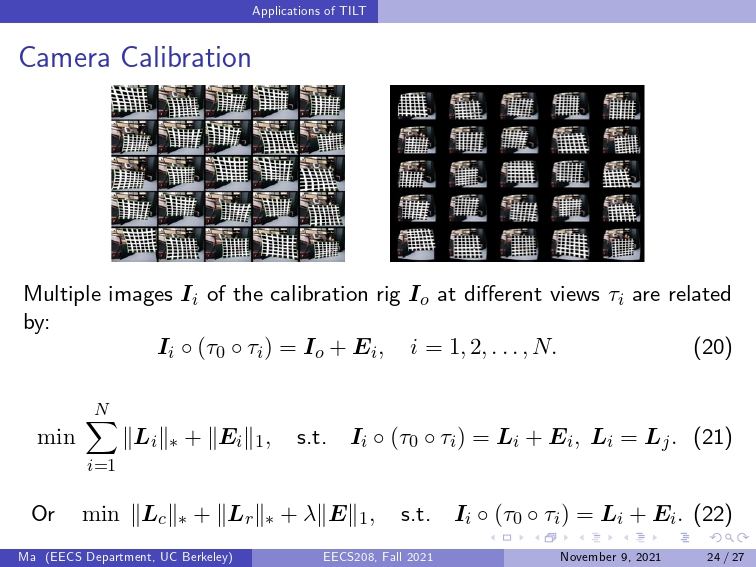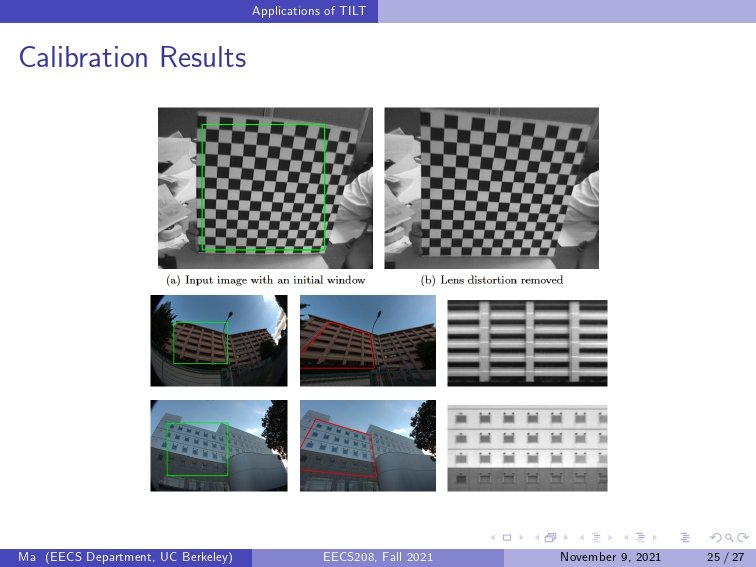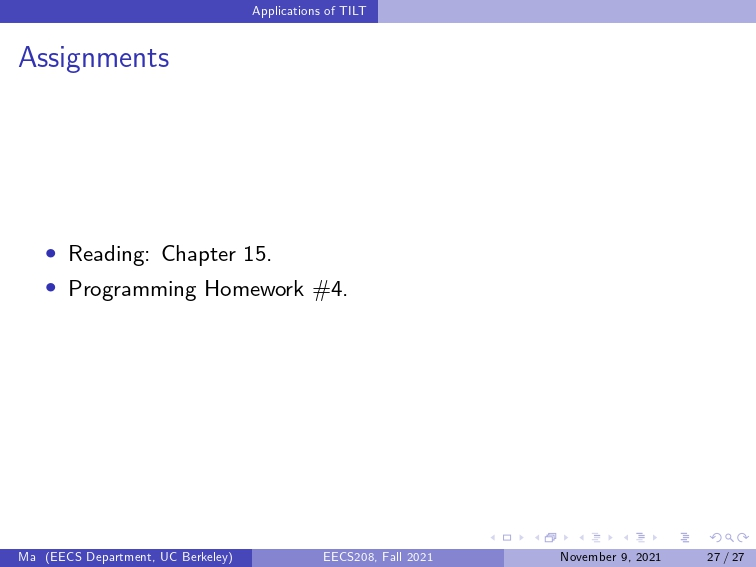...全文
403 回复 打赏 收藏 举报Is local dominant orientation necessary for the classification of rotation invariant texture?
Extracting local rotation invariant features is a popular method for the classification of rotation invariant texture. To address the issue of local rotation invariance, many algorithms based on anisotropic features were proposed. Usually a dominant orientation is found out first, and then anisotropic feature is extracted by this orientation. To validate whether local dominant orientation is necessary for the classification of rotation invariant texture, in this paper, two isotropic statisticalML基石_12_NonLinearTransformation
quadratic hypothesis nonlinear transform price on nonlinear transform structured hypothesis sets10. 机器学习基石-How can Machine Learn? - Nonlinear Transformation
How can Machine Learn? - Nonlinear Transformation How can Machine Learn? - Nonlinear Transformation 1. Quadratic Hypotheses 2. Nonlinear Transform 3. Price of Nonlinear Transform 4. Structured HypotheTILT: Transform Invariant Low-rank Texture
TILT阅读报告 相关概念调研： lowrankmatrix Suppose that we have a rank-r matrix A of size m x n, where r << min(m,n). In many engineering problems, the entries of the matrix are often corrupted by ...【数字图像处理与应用】Lecture 10 彩色图像处理(1)